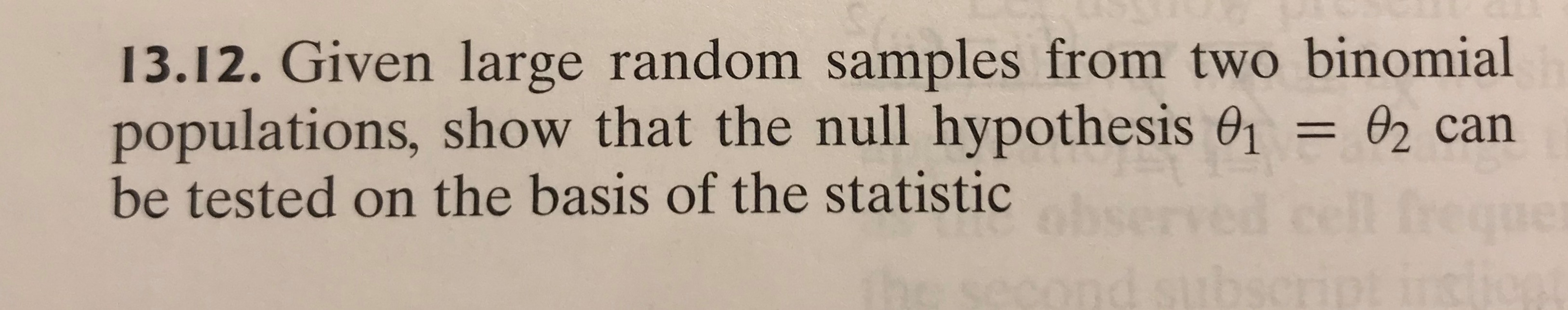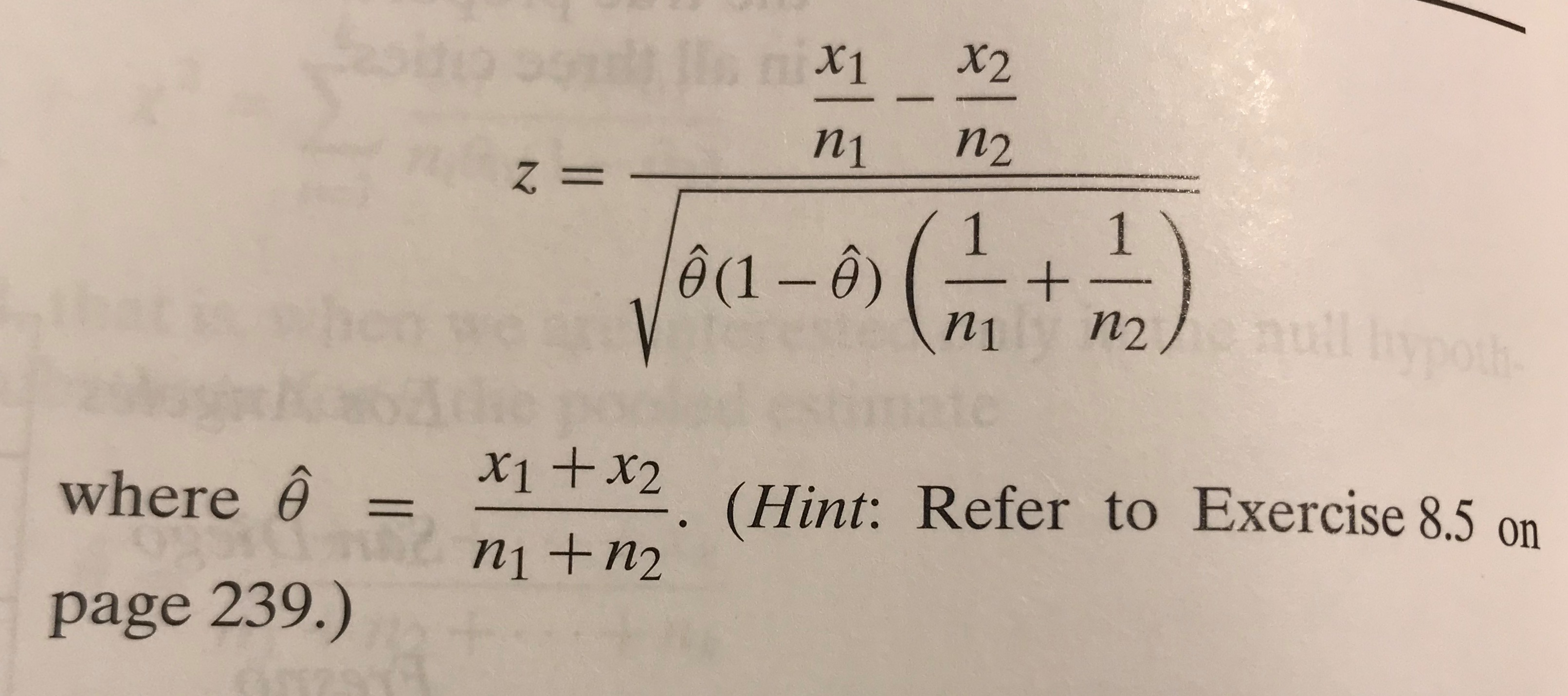# 13.12. Given large random samples from two binomial populations, show that the null hypothesis 01 = 02 can be tested on the basis of the statistic X2 X1 п2 n1 %3| ĝ(1 – Ô) null hypoth n1 П2 X1+x2 where ê = (Hint: Refer to Exercise 8.5 on %3D n +n2 page 239.)

Questionhelp_outlineImage Transcriptionclose13.12. Given large random samples from two binomial populations, show that the null hypothesis 01 = 02 can be tested on the basis of the statistic fullscreenhelp_outlineImage TranscriptioncloseX2 X1 п2 n1 %3| ĝ(1 – Ô) null hypoth n1 П2 X1+x2 where ê = (Hint: Refer to Exercise 8.5 on %3D n +n2 page 239.) fullscreen